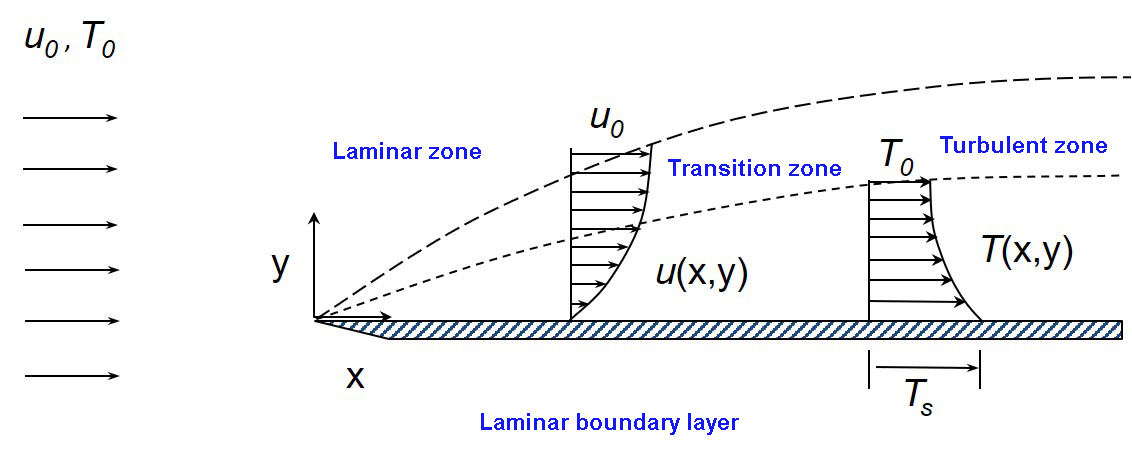--># What is the difference between laminar and turbulent flow?

When a real fluid flows past a solid body or a solid wall, the fluid particles adhere to the boundary and the condition of no-slip occurs. This means that the velocity of fluid close to the boundary will be the same as that of the boundary. If the boundary is stationary, the velocity of the fluid at the boundary layer will be zero. The variation of velocity from zero to free stream velocity in the direction normal to the boundary takes place in a narrow region in the vicinity of a solid boundary. This narrow region of the fluid is called the boundary layer. The theory dealing with the boundary layer flow is called boundary layer theory. A very thin layer of fluid is called the boundary layer.

• Laminar boundary layer:-
For defining the boundary layer consider the flow of liquid, having free stream velocity, over a smooth thin plate that is flat and placed parallel to the direction for a free stream of fluid. consider the flow with zero pressure gradient on one side of the plate, which is stationary.

The velocity of a fluid on the surface of the plate should be equal to the velocity of the plate. But the plate is stationary and hence the velocity of a fluid on the surface of the plate is zero. But at a distance away from the plate, the fluid is having a certain velocity, thus a velocity gradient is set up in the fluid near the surface of the plate. This velocity gradient develops shear resistance, which retards the fluid. The solid surface of the plate and the boundary layer region begin at the sharp leading edge. the boundary layer region increases because the retarded fluid is further retarded, this retardation result in the growth of the boundary layer. Near the leading edge of the surface of the plate, where the thickness is small, the flow in the boundary layer is laminar though the main flow is turbulent.

When a solid body is immersed in a flowing fluid, there is a narrow region of the fluid in the neighborhood of the solid body, where the velocity of fluid varies from zero to free stream velocity. This narrow region of fluid is called the boundary layer.
• Turbulent boundary layer:-
If the length of the plate is more than the thickness of the boundary layer will go increasing in the downstream direction, Then the laminar boundary layer becomes unstable and the motion of fluid within it, is disturbed and irregular which leads to a transition from laminar to the turbulent boundary layer. This short length over which the boundary layer flow changes from laminar to turbulent is called the transition zone. The boundary layer is turbulent and continues to grow thickness. This layer of the boundary is called the turbulent boundary layer.

The remaining fluid, which is outside the boundary layer. the velocity outside the boundary layer is constant and equal to free stream velocity.
• Laminar sub layer:-
This is the region in the turbulent boundary layer zone, adjacent to the solid surface of the plate. In this zone, the velocity variation is influenced only by viscous effects. Though the velocity distribution would be a parabolic curve in the laminar sub-layer zone, in view of the very small thickness we can reasonably assume that velocity variation is linear and so the velocity gradient can be considered constant. Therefore, the shear stress in the laminar sub-layer would be constant and equal to the boundary shear stress.